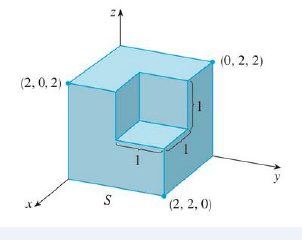Chapter 16, Problem 39RE

Chapter
Section
Textbook Problem

Find ∬S F · n dS, where F(x, y, z) = x i + y j + z k and S is the outwardly oriented surface shown in the figure (the boundary surface of a cube with a unit corner cube removed).To determine

To find: The value of SFndS , where F(x,y,z)=xi+yj+zk and S is the outwardly oriented surface.

Explanation

Given data:

The vector field is F(x,y,z)=xi+yj+zk , where S is the outwardly oriented surface.

Formula used:

Write the expression to find flux of the vector field F(x,y,z) across the surface S .

SFndS=EdivFdV (1)

Here,

E is the solid region.

Write the expression to find divergence of vector field F(x,y,z)=Pi+Qj+Rk .

divF=xP+yQ+zR (2)

Write the expression for volume of cube.

V=a3 (3)

Here,

a is side of the cube.

Find the value of divF .

Substitute x for P , y for Q , and z for R in equation (2),

divF=x(x)+y(y)+z(z)=1+1+1=3

Substitute 3 for divF in equation (1),

SFdS=E3dV

SFdS=3V (4)

Consider the side of full cube is represented by afull .

afull=2

Consider the side of removed portion of the cube is represented by aremoved

Still sussing out bartleby?

Check out a sample textbook solution.

See a sample solution

The Solution to Your Study Problems

Bartleby provides explanations to thousands of textbook problems written by our experts, many with advanced degrees!

Get Started

In Problems 7-34, perform the indicated operations and simplify. 22.

Mathematical Applications for the Management, Life, and Social Sciences

Prove Equation 4.

Single Variable Calculus: Early Transcendentals

For f(x)=xx+1,f(x)=. a) 1 b) x2(x+1)2 c) 1(x+1)2 d) 1(x+1)2

Study Guide for Stewart's Single Variable Calculus: Early Transcendentals, 8th

Explain the difference between passive and active deception.

Research Methods for the Behavioral Sciences (MindTap Course List)